AP State Board Syllabus AP SSC 10th Class Maths Textbook Solutions Chapter 12 Applications of Trigonometry Ex 12.1 Textbook Questions and Answers.

## AP State Syllabus SSC 10th Class Maths Solutions 12th Lesson Applications of Trigonometry Exercise 12.1

### 10th Class Maths 12th Lesson Applications of Trigonometry Ex 12.1 Textbook Questions and Answers

Question 1.
A tower stands vertically on the ground. From a point which is 15 meter away from the foot of the tower, the angle of elevation of the top of the tower is 45°. What is the height of the tower?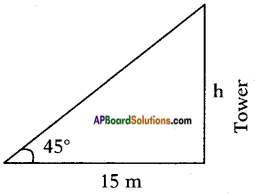Let the height of the tower = h m
Distance of the point of observation from the foot of the tower =15 cm.
Angle of elevation of the top of the tower = 45°
From the figure tan θ = $$\frac{\text { opp. side }}{\text { adj. side }}$$
tan 45° = $$\frac{h}{15}$$
⇒ 1 = $$\frac{h}{15}$$
∴ h = 1 × 15 = 15 mQuestion 2.
A tree breaks due to storm and the broken part bends so that the top of the tree touches the ground by making 30° angle with the ground. The distance between the foot of the tree and the top of the tree on the ground is 6 m. Find the height of the tree before falling down.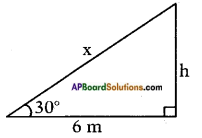Distance between the foot of tree and the point of contact of the top of the tree on the ground = 6 cm.
Let the length of the remaining part be = h m.
Let the length of the broken part be = x m.
Angle made by the broken part with the ground = 30°.
From the figure
tan 30° = $$\frac{h}{6}$$
⇒ $$\frac{1}{\sqrt{3}}$$ = $$\frac{h}{6}$$
∴ h = $$\frac{6}{\sqrt{3}}=\frac{3 \times 2}{\sqrt{3}}$$ = 2√3 m
Also cos 30° = $$\frac{6}{x}$$
⇒ $$\frac{\sqrt{3}}{2}$$ = $$\frac{6}{x}$$
⇒ x = $$\frac{6 \times 2}{\sqrt{3}}$$ = $$\frac{3 \times 2 \times 2}{\sqrt{3}}$$ = 4√3
∴ Height of the tree = broken part + remaining part
= x + h
= 2√3 + 4√3 = 6√3 m
= 6 × 1.732
≃ 10.392 m.Question 3.
A contractor wants to set up a slide for the children to play in the park. He wants to set it up at the height of 2 m and by making an angle of 30° with the ground. What should be the length of the slide?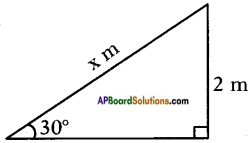Height of slide = 2 m
Let the length of the slide = x m.
Angle made by the slide with the ground = 30°
From the figure
sin 30° = $$\frac{2}{x}$$
⇒ $$\frac{1}{2}$$ = $$\frac{2}{x}$$
⇒ x = 2 × 2 = 4 m
Length of the slide = 4 m.

Question 4.
Length of the shadow of a 15 meter high pole is 5√3 meters at 7 o’clock in the morning. Then, what is the angle of elevation of the Sun rays with the ground at the time?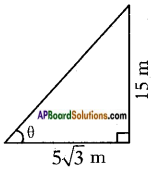Height of the pole = 15 m
Length of the shadow = 5√3 m
Let the angle of elevation be ‘θ’.
Then from the figure
tan θ = $$\frac{15}{5 \sqrt{3}}=\frac{5 \times \sqrt{3} \times \sqrt{3}}{5 \times \sqrt{3}}$$ = √3
tan θ = √3 = tan 60°
∴ θ = 60°
∴ Angle of elevation of Sun rays with the ground = 60°.Question 5.
You want to erect a pole of height 10 m with the support of three ropes. Each rope has to make an angle 30° with the pole. What should be the length of the rope?
Height of the pole = 10 m
Let the length of each rope = x
Angle made by the rope with the pole = 30°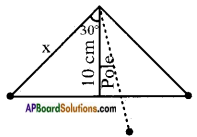From the figure
cos 30° = $$\frac{10}{x}$$
$$\frac{\sqrt{3}}{2}$$ = $$\frac{10}{x}$$
⇒ x = $$\frac{10 \times 2}{\sqrt{3}}=\frac{20}{\sqrt{3}}$$
∴ Length of each rope = $$\frac{20}{\sqrt{3}}$$m
= 11.546 m.

∴ Total length of the rope = 3 × $$\frac{20}{\sqrt{3}}$$
= 20√3
= 20 × 1.732
≃ 34.64 m.

Question 6.
Suppose you are shooting an arrow from the top of a building at a height of 6 m to a target on the ground at an angle of depression of 60°. What is the distance between you and the object?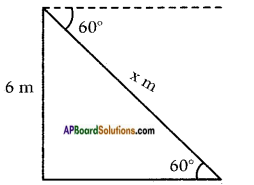Height of the building = 6 m
Angle of depression = Angle of elevation at the ground = 60°
Let the distance of the target from the shooting point = x m
Then from the figure
sin 60° = $$\frac{6}{x}$$
$$\frac{\sqrt{3}}{2}$$ = $$\frac{6}{x}$$
⇒ x = $$\frac{6 \times 2}{\sqrt{3}}=\frac{2 \times \sqrt{3} \times \sqrt{3} \times 2}{\sqrt{3}}$$ = 4√3
∴ Distance = 4√3 m or
4 × 1.732 = 6.928 m.Question 7.
An electrician wants to repair an electric connection on a pole of height 9 m. He needs to reach 1.8 m below the top of the pole to do repair work. What should be the length of the ladder which he should use, when he climbs it at an angle of 60° with the ground? What will be the distance between foot of the ladder and foot of the pole?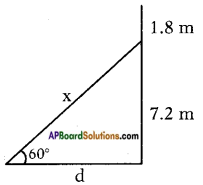Height of the pole = 9m
Height of the point from the ground where he reaches the pole = 9 – 1.8 = 7.2 m
Angle of elevation = 60°
Angle of depression = Angle of elevation at the ground = 60°
Let the distance of the target from the shooting point = x m
Then from the figure
sin 60° = $$\frac{7.2}{x}$$
$$\frac{\sqrt{3}}{2}$$ = $$\frac{7.2}{x}$$
⇒ x = $$\frac{7.2 \times 2}{\sqrt{3}}=\frac{3 \times 2.4 \times 2}{\sqrt{3}}=\frac{\sqrt{3} \times \sqrt{3} \times 4.8}{\sqrt{3}}$$
⇒ x = 1.732 × 4.8
≃ 8.31 m
Also tan 60° = $$\frac{7.2}{d}$$
√3 = $$\frac{7.2}{d}$$
⇒ d = $$\frac{7.2}{\sqrt{3}}=\frac{2.4 \times 3}{\sqrt{3}}$$ = 2.4 × √3 = 2.4 × 1.732
∴ d ≃ 4.1568 m

Question 8.
A boat has to cross a river. It crosses the river by making an angle of 60° with the bank of the river due to the stream of the river and travels a distance of 600 m to reach the another side of the river. What is the width of the river?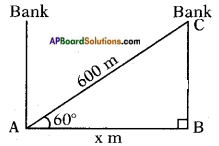Let the width of the river = AB = x m
Angle made by the boat = 60°
Distance travelled = AC = 600 m
From the figure
cos 60° = $$\frac{x}{600}$$
$$\frac{1}{2}$$ = $$\frac{x}{600}$$
⇒ x = $$\frac{600}{2}$$ = 300 m.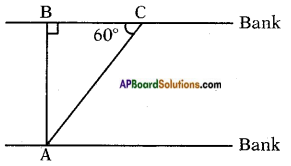In the figure
A = Boat’s place
C = Reach place of another side (or) Point of observation.
AC = Travelling distance of the boat ∠AC = 60°
AB = width of the river AB
In △ABC, sin 60° = $$\frac{AB}{AC}$$
⇒ $$\frac{\sqrt{3}}{2}$$ = $$\frac{AB}{600}$$
⇒ AB = 600 × $$\frac{\sqrt{3}}{2}$$ = 300√3Question 9.
An observer of height 1.8 m is 13.2 m away from a palm tree. The angle of elevation of the top of the tree from his eyes is 45°. What is the height of the palm tree?
Height of the observer = 1.8 m
Distance of the observer from the palm tree = 13.2 m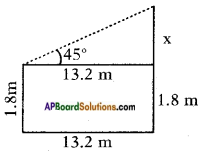From the figure
tan 45° = $$\frac{x}{13.2}$$
⇒ 1 = $$\frac{x}{13.2}$$
⇒ x = 13.2 m
∴ Height of the palm tree = 13.2 + 1.8 = 15 m.

Question 10.
In the given figure, AC = 6 cm, AB = 5 cm and ∠BAC = 30°. Find the area of the triangle.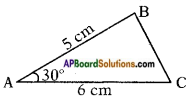Answer: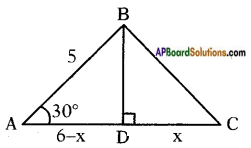Draw a perpendicular BD to AC
∴ BD ⊥ AC
Now let AD = 6 – x and DC = x
Given AB = 5 cm and ∠BAD = 30° then in △ABD
sin 30° = $$\frac{BD}{AB}$$ = $$\frac{BD}{5}$$ = $$\frac{1}{2}$$
⇒ BD = $$\frac{5}{2}$$ = 2.5 cm
and cos 30° = $$\frac{AD}{AB}$$ = $$\frac{6-x}{5}$$ = $$\frac{\sqrt{3}}{2}$$
⇒ 6 – x = $$\frac{5 \sqrt{3}}{2}$$
⇒ x = 6 – $$\frac{5 \sqrt{3}}{2}$$ = 6 – $$\frac{5(1.732)}{2}$$
∴ x = 1.67
∴ Area of △ABC = $$\frac{1}{2}$$bh
= $$\frac{1}{2}$$ × AC × BD
= $$\frac{1}{2}$$ × 6 × 2.5
= 7.5 cm2